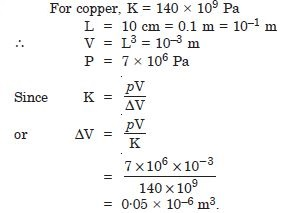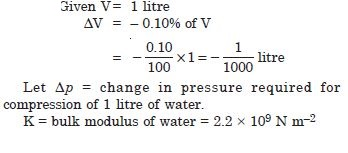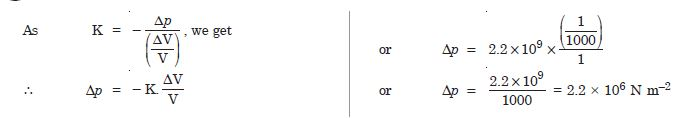# NCERT Solutions for Class 11 Physics Chapter 9 - Mechanical Properties of Solids

##### Question 1: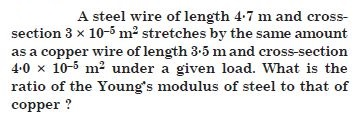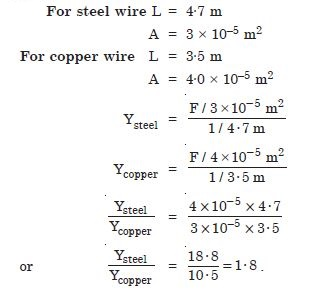##### Question 2:

The given figure shows the strain– stress curve for a given material. What are
(a) Young’s modulus and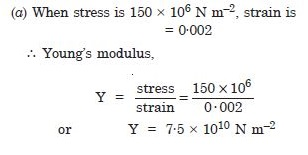##### Question 3:

The given figure shows the strain– stress curve for a given material. What are
(b) approximate yield
strength for this material ?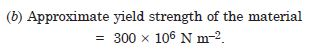##### Question 4:

The stress-strain graphs for materials A and B for two materials are shown in Fig.9.2 (The graphs are to the same scale).
Which material has greater Young’s modulus ?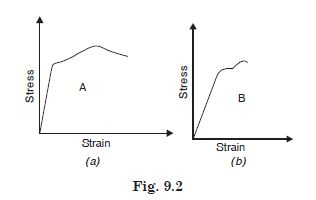A material has greater Young’s modulus.

##### Question 5:

The stress-strain graphs for materials A and B for two materials are shown in Fig.9.2 (The graphs are to the same scale).
Which of the two is the stronger material ?Strength of a material is determined by the amount of stress required to cause fracture. Material (A) is stronger than (B).

##### Question 6:

Read the statement below carefully and state, with reason, if it is true or false ?
The Young’s modulus of elasticity of rubber is greater than that of steel.

False, because in case of steel for same stress, strain is less.

##### Question 7:

Read the statement below carefully and state, with reason, if it is true or false ?
The stretching of a coil spring is determined by its shear modulus.

True, because stress produces, relative displacement between threads of coil.

##### Question 8:

Two wires of diameter 0·25 cm, one made of steel and the other made of brass and loaded as shown in the figure 9.3. The unloaded length of steel wire is 1·5 m and that of brass wire is 1·0 m. Compute the elongations of the steel and the brass wires.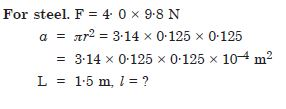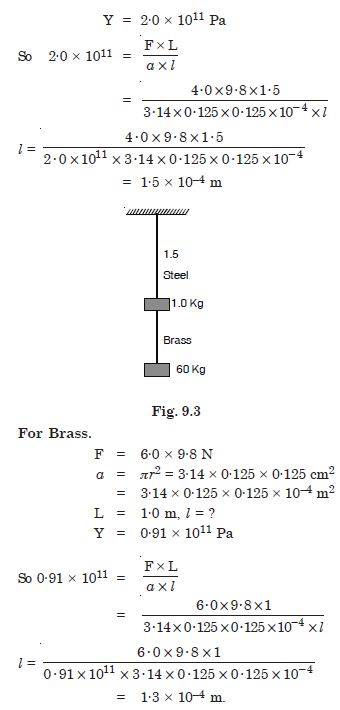##### Question 9:

The edges of an aluminium cube are 10 cm long. One face of the cube is firmly fixed to a vertical wall. A mass of 1000 kg is then attached to the opposite face of the cube. The shear modulus of aluminium is 25 GPa. What is vertical deflection of this face ? (1Pa = 1 N m–2)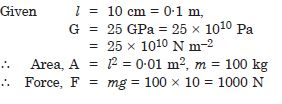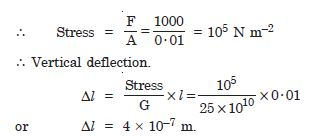##### Question 10:

Four identical hollow cylindrical columns of steel support a big structure of mass 50000 kg the inner and outer radii of each column are 30 cm and 60 cm respectively. Assume the load distribution to be uniform. Calculate the compressional strain of each column. Y for steel = 2·0 × 10–11 Pa.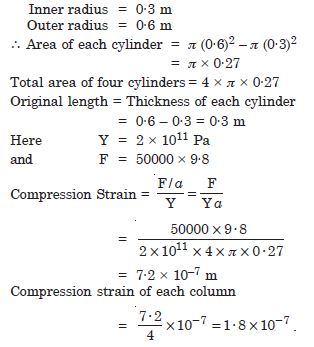##### Question 11:

A piece of copper having cross-section of 15·2 mm × 19·1 mm is pulled in tension with 44,500 N force, producing only elastic deformation. Calculate the resulting strain.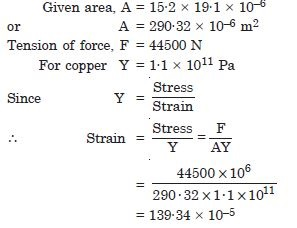##### Question 12:

A steel cable with a radius of 1·5 cm supports a chairlift at a ski area. If the maximum stress is not to exceed 108 N m–2, what is the maximum load the cable can support ?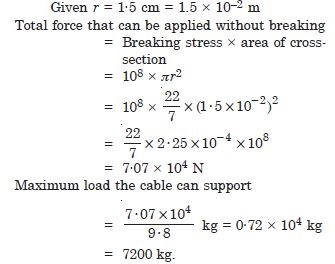##### Question 13:

A rigid bar of mass 15 kg is supported symmetrically by three wires each 2 m long. Those at each end are of copper and the middle one is of iron. Determine the ratios of their diameters if each is to have the same tension.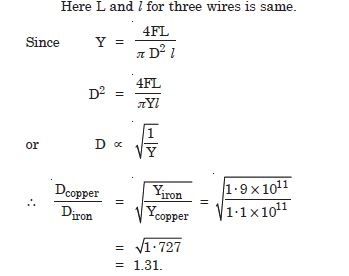##### Question 14:

A 14·5 kg mass, fastened to the end of a steel wire of unstretched length 1 m, is whirled in a vertical circle with an angular velocity of 2 rev/s at the bottom of the circle. The cross-sectional area of the wire is 0·065 cm2. Calculate the elongation of the wire when mass is at the lowest point of its path.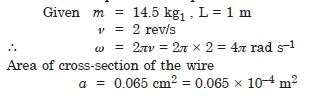##### Question 15:

Compute the bulk modulus of water from the following data : initial volume = 100.0 litre, pressure increase = 100.0 atm (1 atm = 1.013 × 105 Pa), Final volume = 100.5 litre. Compare the bulk modulus of water with that of air (at constant temperature). Explain in simple terms why the ratio is so large ?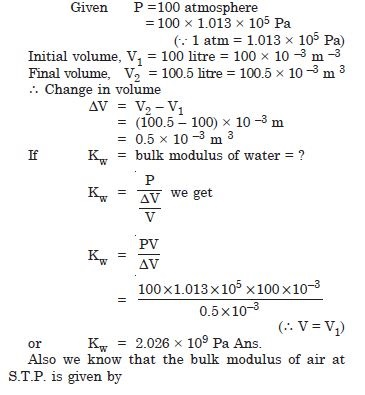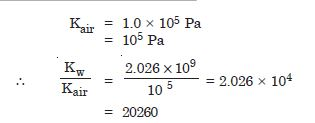The ratio is too large. This is due to the fact that the strain for air is much larger than for water at the same temperature. In other words the intermolecular distances in case of liquids are very small as compared to the corresponding distances in the case of gases. Hence there are larger inter atomic forces in liquids than in gases

##### Question 16: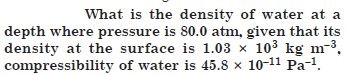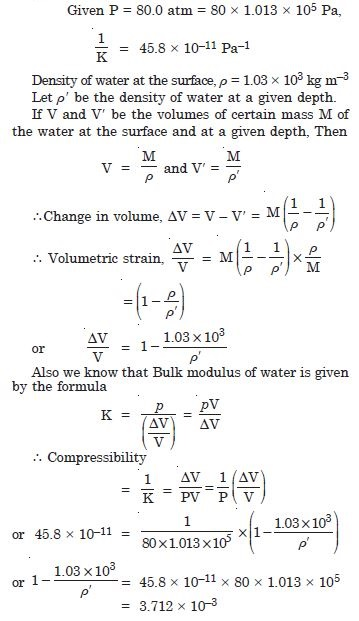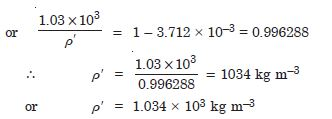##### Question 17:

Compute the fractional change in volume of a glass slab, when subjected to a hydraulic pressure of 10 atm.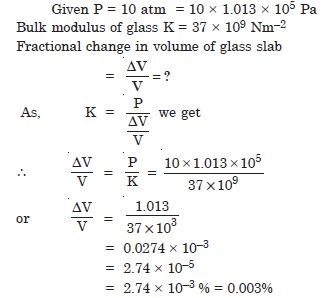##### Question 18:

Determine the volume contraction of a solid copper cube, 10 cm on an edge, when subjected to a hydraulic pressure of 7 × 106 Pa.